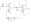# Electronic Circuits July 2008

Note : Answer any FIVE fall questions.

1  a. For the sketch shown in fig.Ql(a) below,Vj varies from 0 to 150V. Sketch the output voltage V0 to the same time scale as the input voltage. Assume diodes to be ideal.

b.  Explain the operation of full wave voltage doubler circuit.

c.  A full wave rectifier circuit is fed from a secondary center tapped transformer. The rms voltage from either end of secondary to center tap is 30V if diode resistance Rf = 2Ω, half secondary resistance Rs=8Ω and load RL= 1kΩ, calculate efficiency of rectification.

2 a. Discuss the causes for bias instability in a transistor,

b.  With neat circuit diagram, explain how compensation for Vbe can be obtained using diode in emitter circuit of a transistor.

c.  A Ge transistor used in self biased circuit has Vcc = 20V, Rc ” 2k, operating point VCe = 10V and Ic — 4mA, and p – 50. Calculate Ri, R2 and RE if stability factor S = 5IC /5Ico=10 is desired.

3 a. Discuss the biasing techniques used for linear integrated circuits.

b. Derive the expression for Ay Ai Rjn and Ro of an emitter follower.

4 a. Derive the expression for transistor transcon ductance gm and input conductance, gbc in the case of transistor.

b.  State and prove Miller’s theorem.

5 a. Derive an expression for input and output resistance of voltage series feedback amplifier.

b.  An amplifier with negative feedback has a voltage gain of 120. It is found that without feedback, an input signal of 60mV is required to produce a particular output, where as with feedback the input signal must be 0.5V to get the same output. Find the Av and J3 of the amplifier.

c.  Mention the advantages of negative feedback.

6  a. Derive an expression for the maximum conversion efficiency of a class B push – pull amplifier.

b.  Calculate the input power, output power and efficiency of the amplifier in the figure Q6(b) shown for an input voltage in a base current of 10mA peak. Also calculate the power dissipated by the transistor.Fig-6(b)

7  a. Draw the circuit and explain how to measure the CMRR of an OP-amp.

b.  Find output voltage V0 in terms of Vi and V2 for the op-amp circuit shown in fig. 7(b). Assume ideal op-amps.Fig. 7(b)

c.  Design a Schmitt trigger whose Vlt and Vur are ± 5V. Draw waveforms. Take LM741 with Vsat = ± 13.5V and supply voltage ± 15V.

8 a. Draw and explain the working of positive clamper.

b.  Explain the working of D/A converter [Binary weighted resistors] with neat sketch.

c.  Give the circuit schematic off 555 timer connected as an a stable multivibrator. Describe its operation.